# What is non-trivial solution to linear algebra

## Ψ The IT side

### Solution criteria for linear systems of equations

To determine the number of solutions to a linear equation, exist Solution criteria:

Homogeneous systems of linear equations can have either the trivial solution or multiple solutions.

• Trivial solution:
The trivial solution is this Zero vector; this means that you can only satisfy all equations if you set all variables to zero.
This case occurs when the homogeneous system of linear equations can be brought into row step form in such a way that a zero is added in each row from top to bottom and we have just as many rows as columns in the matrix1.3. Example of a system of equations with a matrix in row level form, which says that there is only the trivial solution: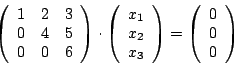With this system of equations follows from the last line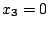. Then follows from the second line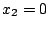and finally from the first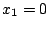, which is why we only get the zero vector as a solution.
With a homogeneous system of linear equations we can test whether vectors are linearly dependent or independent of one another. We write the vectors into the matrix as column vectors. If the homogeneous system of linear equations obtained in this way only has the trivial solution, the vectors are linearly independent of one another.

• Multiple solutions: The homogeneous system of linear equations has several solutions if it free variables gives. A free variable is a variable that is not determined by solving the system of equations. We can choose such a variable arbitrarily and thus get any number of solutions for the system of equations. These solutions span a vector space.
How do we now know whether the system of equations has free variables? Free variables are always available when there are fewer equations in the system than there are variables. Example: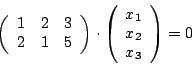There are also systems of linear equations where it is not so obvious that there are fewer equations than variables. In these systems of equations, by bringing the system to row level form, we recognize that a Zero line exists. In homogeneous systems of linear equations, we can simply cross out such zero lines (be careful! This does not work in inhomogeneous systems, as otherwise we could destroy the fact that there is no solution.). If there are more variables than equations left after deleting, we have a system of equations with free variables.
If, when solving a homogeneous linear system of equations, in which the matrix consists of column vectors, whose linear independence from one another is to be checked, it emerges that this system of equations has several solutions, then the vectors are linearly dependent on one another.

Inhomogeneous Systems of equations cannot have a solution, exactly one or more solutions:
• No solution:
An inhomogeneous system of linear equations has no solution if the coefficients of one of the equations are zero, the associated one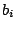but is not null: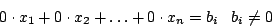Such an equation is called degenerate. Degenerate equations cannot be fulfilled and thus the entire system of equations cannot be fulfilled, which is why we have no solution.

• Exactly one solution:
An inhomogeneous system of equations has exactly one solution if the associated homogeneous system of equations - the associated homogeneous system of equations is generated by allbe set to zero - only the trivial solution has.
In order to calculate the solution, we bring the inhomogeneous linear system of equations into line-level form, as we have also done above, whereby here we use the(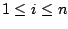) have to take into account.
An inhomogeneous system of equations can be used to check whether a vector can be generated as a linear combination of other vectors. There is a solution if and only ifcan be generated by linear combination of the column vectors. If there is no solution, one can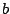do not combine from the column vectors. If there is one or even several solutions, this is possible.

• Multiple solutions:
As with homogeneous linear systems of equations, free variables can also appear in inhomogeneous systems. These exist again when you have more variables than equations. Zero lines can also be deleted in inhomogeneous systems, wherever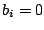. However, the degenerate equations must not be deleted.